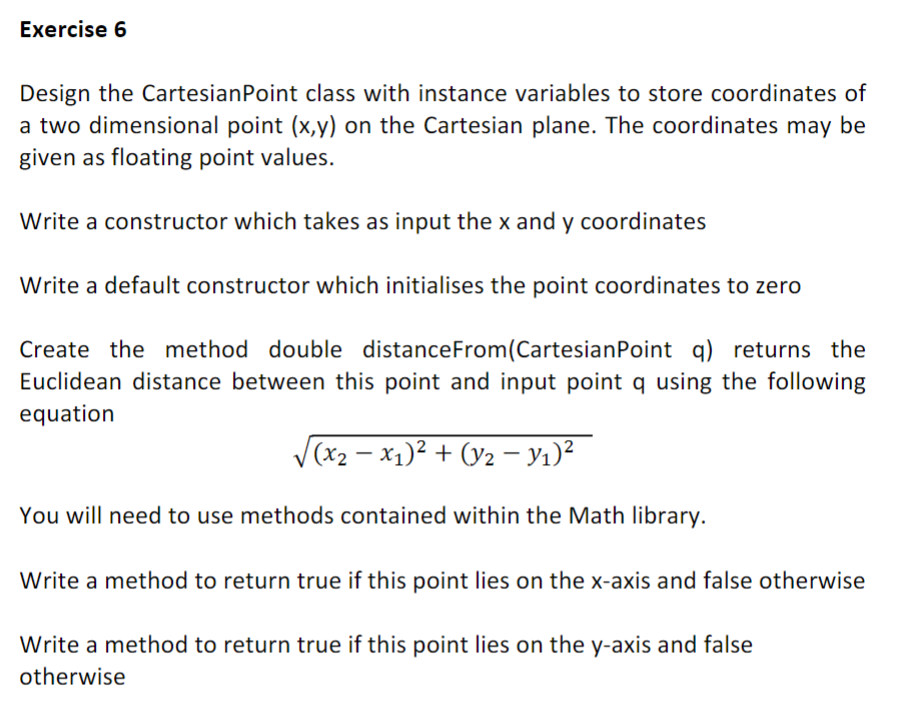# Question please answer in java language Exercise 6 Design the CartesianPoint class with instance variables to store coordinates of a two dimensional point (x,y) on the Cartesian plane. The coordinates may be given as floating point values. Write a constructor which takes as input the x and y coordinates Write a default constructor which initialises the point coordinates to zero Create the method double distance From(CartesianPoint q) returns the Euclidean distance between this point and input point q using the following equation (x2 – x1)2 + (y2 – yı)2 You will need to use methods contained within the Math library. Write a method to return true if this point lies on the x-axis and false otherwise Write a method to return true if this point lies on the y-axis and false otherwise7XPMIA The Asker · Computer Science